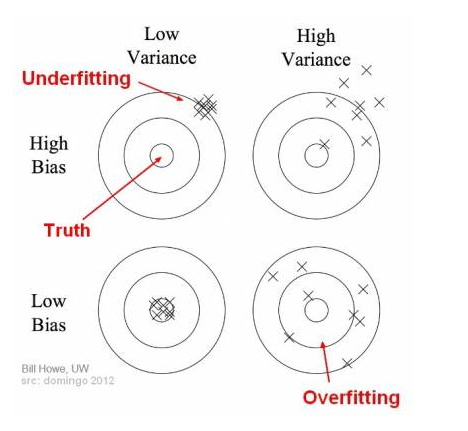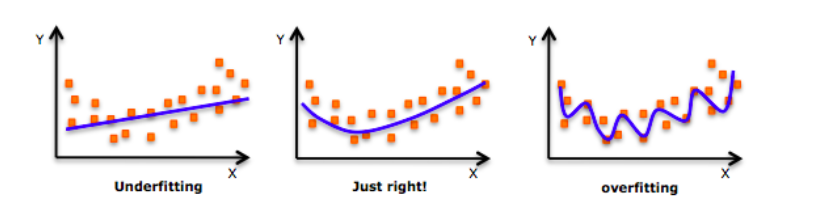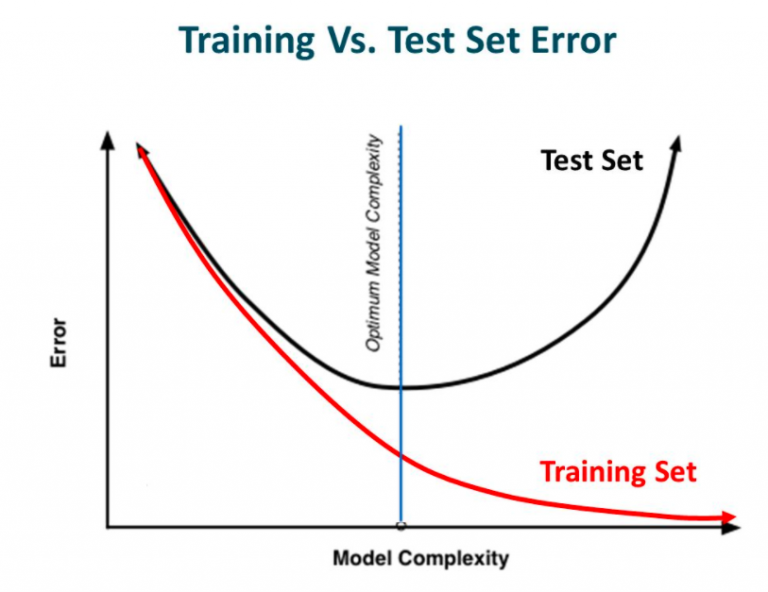# Overfitting Challenge

Overfitting is a problem in machine learning in general, not just in neural networks. The problem is inherent in the way machine learning models are developed: A set of "training data" is used to "train" the model. The goal is to have a model that can then be used on data that hasn't been seen before. Over-fitting refers to the problem of having the model trained to work so well on the training data that it starts to work more poorly on data it hasn't seen before. There are a number of techniques to mitigate or prevent over-fitting. | Deep Learning Course Wiki

• Bias is the simplifying assumptions made by the model to make the target function easier to approximate.
• Variance is the amount that the estimate of the target function will change given different training data.

Trade-off is tension between the error introduced by the bias and the variance. Jason BrownleeWhenever we discuss model prediction, it’s important to understand prediction errors (bias and variance). There is a tradeoff between a model’s ability to minimize bias and variance... To build a good model, we need to find a good balance between bias and variance such that it minimizes the total error.Understanding the Bias-Variance Tradeoff | Seema Singh